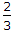# Mechanical Engineering - Hydraulics and Fluid Mechanics

### Exercise :: Hydraulics and Fluid Mechanics - Section 2

6.

The discharge over a rectangular weir, considering the velocity of approach, is (where H1 = Total height of water above the weir = H + Ha H = Height of water, over the crest of the weir, and Ha = Height of water, due to velocity of approach)

 A.Cd x L2g [H1 - Ha] B.Cd x L2g [H13/2 - Ha3/2] C.Cd x L2g [H12 - Ha2] D.Cd x L2g [H15/2 - Ha5/2]

Answer: Option B

Explanation:

No answer description available for this question. Let us discuss.

7.

A flow in which __________ force is dominating over the viscosity is called turbulent flow.

 A. elastic B. surface tension C. viscous D. inertia

Answer: Option D

Explanation:

No answer description available for this question. Let us discuss.

8.

If an incompressible liquid is continuously flowing through a pipe, the quantity of liquid passing per second is different at different sections.

 A. True B. False

Answer: Option B

Explanation:

No answer description available for this question. Let us discuss.

9.

The atmospheric pressure at sea level is

 A. 103 kN/m2 B. 10.3 m of water C. 760 mm of mercury D. all of these

Answer: Option D

Explanation:

No answer description available for this question. Let us discuss.

10.

When the pressure intensity at a point is less than the local atmospheric pressure, then the difference of these two pressures is called vacuum pressure.

 A. Agree B. Disagree

Answer: Option A

Explanation:

No answer description available for this question. Let us discuss.

#### Current Affairs 2021

Interview Questions and Answers